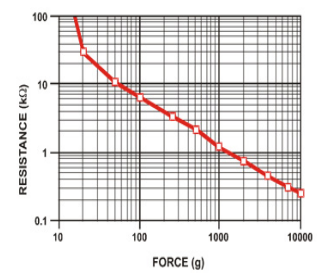# [Force sensor resistance(FSR) sensor] characteristic curve clarification

• Hello all,

I am using Interlink electronics FSR sensors for my project which varies resistance when force is applied, while going through datasheet i came across the characteristic curve of the sensor which is as follows ;So my question is that , Force (g) on x axis is Newton ? or only weight in gram which can be converted into force using F =ma formula ?

Your help in this regard will be highly appreciated.

Regards,

• I'd say grams, but did you try to see what results you get?

• but did you try to see what results you get

I actually applied the load of 5kg on the sensor then got resistance as output.

But i am just confused with this chart at x-axis whether it is weight which can be converted into force using F = ma or it is Force ?

• I would agree the graph shows resistance vs. grams. It looks your 5kg mass should read about .5 ohms. So if you want force (or wieght) multiply 5 x 9.81. The nice thing about this is f=ma is a linear function so you can make a similar graph for resisitance vs. force.

The force value appears to be logarithmic compared to the resistance read this is where the heavy math is, reporting force or mass is a matter of constants. Hopefully the data sheet gives the formula.

• @wallyllama Thankyou for your reply. Actually only voltage conversion formula is given in the data sheet , here is the following link for the data sheet :

But i again thankyou for your time that you clarified my confusion .1

50

7

5

10

19

1

32